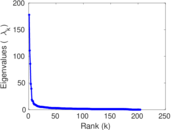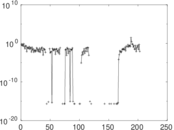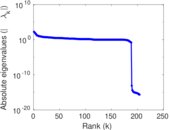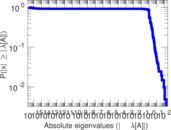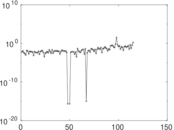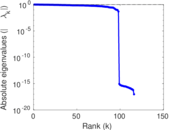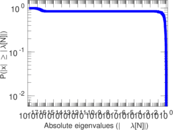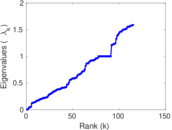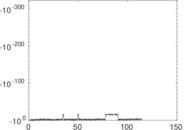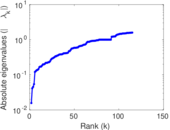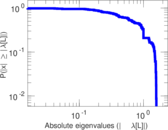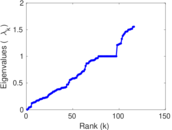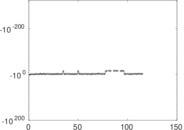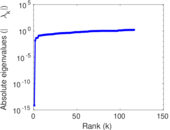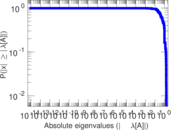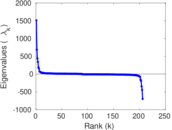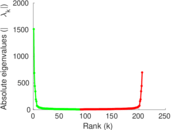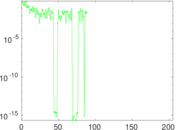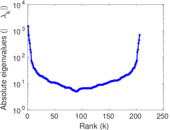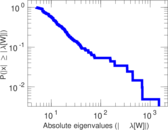# Wikiquote edits (cy)

This is the bipartite edit network of the Welsh Wikisource. It contains users and pages from the Welsh Wikisource, connected by edit events. Each edge represents an edit. The dataset includes the timestamp of each edit.

 Code `qcy` Internal name `edit-cywikisource` Name Wikiquote edits (cy) Data source http://dumps.wikimedia.org/ AvailabilityDataset is available for download Consistency checkDataset passed all tests Category Authorship network Dataset timestamp 2017-10-20 Node meaning User, article Edge meaning Edit Network formatBipartite, undirected Edge typeUnweighted, multiple edges Temporal dataEdges are annotated with timestamps

## Statistics

 Size n = 1,511 Left size n1 = 206 Right size n2 = 1,305 Volume m = 3,417 Unique edge count m̿ = 1,696 Wedge count s = 168,901 Claw count z = 22,105,621 Cross count x = 2,507,874,854 Square count q = 4,572 4-Tour count T4 = 717,752 Maximum degree dmax = 1,454 Maximum left degree d1max = 1,454 Maximum right degree d2max = 193 Average degree d = 4.522 83 Average left degree d1 = 16.587 4 Average right degree d2 = 2.618 39 Fill p = 0.006 308 82 Average edge multiplicity m̃ = 2.014 74 Size of LCC N = 1,246 Diameter δ = 14 50-Percentile effective diameter δ0.5 = 3.720 24 90-Percentile effective diameter δ0.9 = 5.972 61 Median distance δM = 4 Mean distance δm = 4.509 19 Gini coefficient G = 0.720 559 Balanced inequality ratio P = 0.221 100 Left balanced inequality ratio P1 = 0.125 549 Right balanced inequality ratio P2 = 0.308 458 Relative edge distribution entropy Her = 0.798 083 Power law exponent γ = 4.890 37 Tail power law exponent γt = 2.601 00 Tail power law exponent with p γ3 = 2.601 00 p-value p = 0.013 000 0 Left tail power law exponent with p γ3,1 = 1.841 00 Left p-value p1 = 0.492 000 Right tail power law exponent with p γ3,2 = 3.681 00 Right p-value p2 = 0.082 000 0 Degree assortativity ρ = −0.182 806 Degree assortativity p-value pρ = 3.276 97 × 10−14 Spectral norm α = 177.798 Algebraic connectivity a = 0.015 683 4 Spectral separation |λ1[A] / λ2[A]| = 1.603 00 Controllability C = 1,103 Relative controllability Cr = 0.745 774

## Plots

### Fruchterman–Reingold graph drawing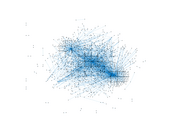### Degree distribution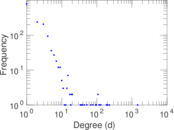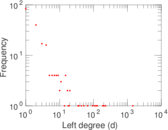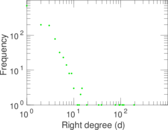### Cumulative degree distribution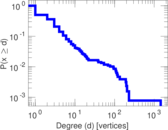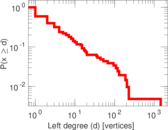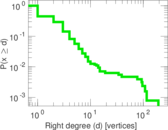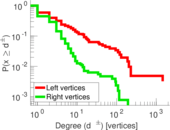### Lorenz curve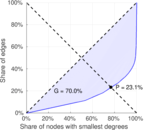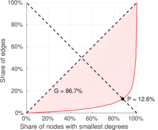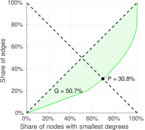### Spectral distribution of the adjacency matrix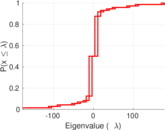### Spectral distribution of the normalized adjacency matrix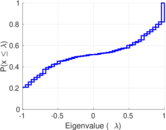### Spectral distribution of the Laplacian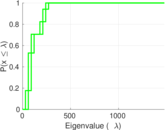### Spectral graph drawing based on the adjacency matrix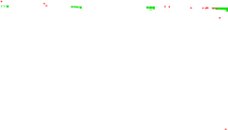### Spectral graph drawing based on the Laplacian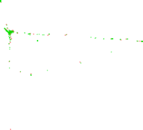### Spectral graph drawing based on the normalized adjacency matrix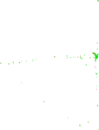### Degree assortativity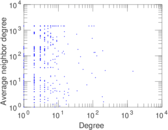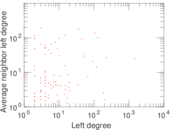### Zipf plot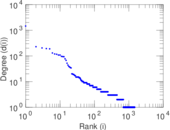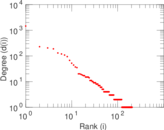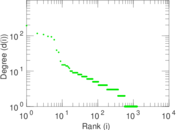### Hop distribution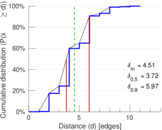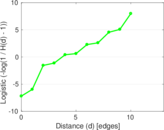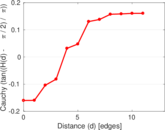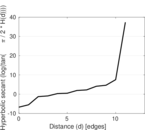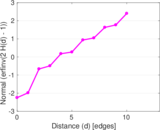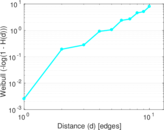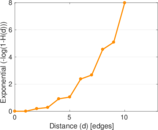### Double Laplacian graph drawing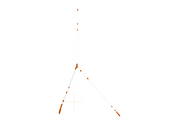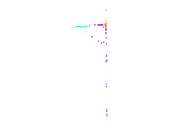### Delaunay graph drawing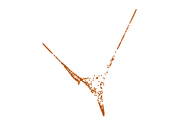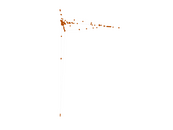### Edge weight/multiplicity distribution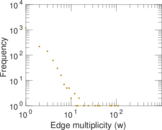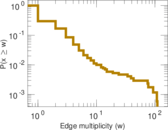### Temporal distribution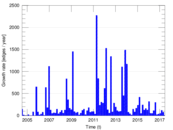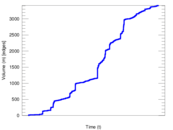### Temporal hop distribution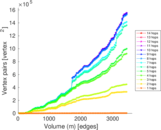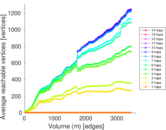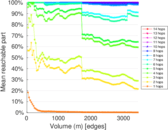### Diameter/density evolution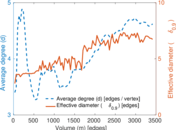### Matrix decompositions plots